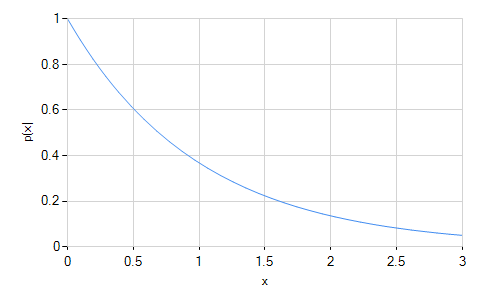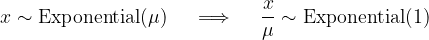﻿ExponentialDistribution Class# ExponentialDistribution Class

Represents an exponential distribution.Inheritance Hierarchy

Namespace:  Meta.Numerics.Statistics.Distributions
Assembly:  Meta.Numerics (in Meta.Numerics.dll) Version: 4.1.4Syntax
`public sealed class ExponentialDistribution : ContinuousDistribution`

The ExponentialDistribution type exposes the following members.Constructors
NameDescriptionExponentialDistribution
Initializes a new standard exponential distribution.ExponentialDistribution(Double)
Initializes a new exponential distribution with the given mean.
TopProperties
NameDescriptionExcessKurtosis
Gets the excess kurtosis of the distribution.
(Overrides UnivariateDistributionExcessKurtosis.)Mean
Gets the mean of the distribution.
(Overrides UnivariateDistributionMean.)Median
Gets the median of the distribution.
(Overrides ContinuousDistributionMedian.)Skewness
Gets the skewness of the distribution.
(Overrides UnivariateDistributionSkewness.)StandardDeviation
Gets the standard deviation of the distribution.
(Overrides UnivariateDistributionStandardDeviation.)Support
Gets the interval over which the distribution is non-vanishing.
(Overrides ContinuousDistributionSupport.)Variance
Gets the variance of the distribution.
(Inherited from UnivariateDistribution.)
TopMethods
NameDescriptionCentralMoment
Computes a central moment of the distribution.
(Overrides ContinuousDistributionCentralMoment(Int32).)Cumulant
Computes a cumulant of the distribution.
(Overrides UnivariateDistributionCumulant(Int32).)Equals
Determines whether the specified object is equal to the current object.
(Inherited from Object.)ExpectationValue
Computes the expectation value of the given function.
(Inherited from ContinuousDistribution.)FitToSample
Computes the exponential distribution that best fits the given sample.GetHashCode
Serves as the default hash function.
(Inherited from Object.)GetRandomValue
Generates a random variate.
(Overrides ContinuousDistributionGetRandomValue(Random).)GetRandomValues
Generates the given number of random variates.
(Inherited from ContinuousDistribution.)GetType
Gets the Type of the current instance.
(Inherited from Object.)Hazard
Computes the hazard function.
(Overrides ContinuousDistributionHazard(Double).)InverseLeftProbability
Returns the point at which the cumulative distribution function attains a given value.
(Overrides ContinuousDistributionInverseLeftProbability(Double).)InverseRightProbability
Returns the point at which the right probability function attains the given value.
(Overrides ContinuousDistributionInverseRightProbability(Double).)LeftProbability
Returns the cumulative probability to the left of (below) the given point.
(Overrides ContinuousDistributionLeftProbability(Double).)ProbabilityDensity
Returns the probability density at the given point.
(Overrides ContinuousDistributionProbabilityDensity(Double).)RawMoment
Computes a raw moment of the distribution.
(Overrides ContinuousDistributionRawMoment(Int32).)RightProbability
Returns the cumulative probability to the right of (above) the given point.
(Overrides ContinuousDistributionRightProbability(Double).)ToString
Returns a string that represents the current object.
(Inherited from Object.)
TopRemarks

An exponential distribution falls off exponentially in the range from zero to infinity. It is a one-parameter distribution, determined entirely by its rate of fall-off.The exponential distribution describes the distribution of decay times of radioactive particles.

An exponential distribution with mean one is called a standard exponential distribution. Any exponential distribution can be converted to a standard exponential by re-parameterizing the data into "fractions of the mean," i.e. z = x / μ.Processes resulting in events that are exponentially distributed in time are said to be "ageless" because the hazard function of the exponential distribution is constant. The Weibull distribution (WeibullDistribution) is a generalization of the exponential distribution for which the hazard function changes (usually by increasing) with time.See Also